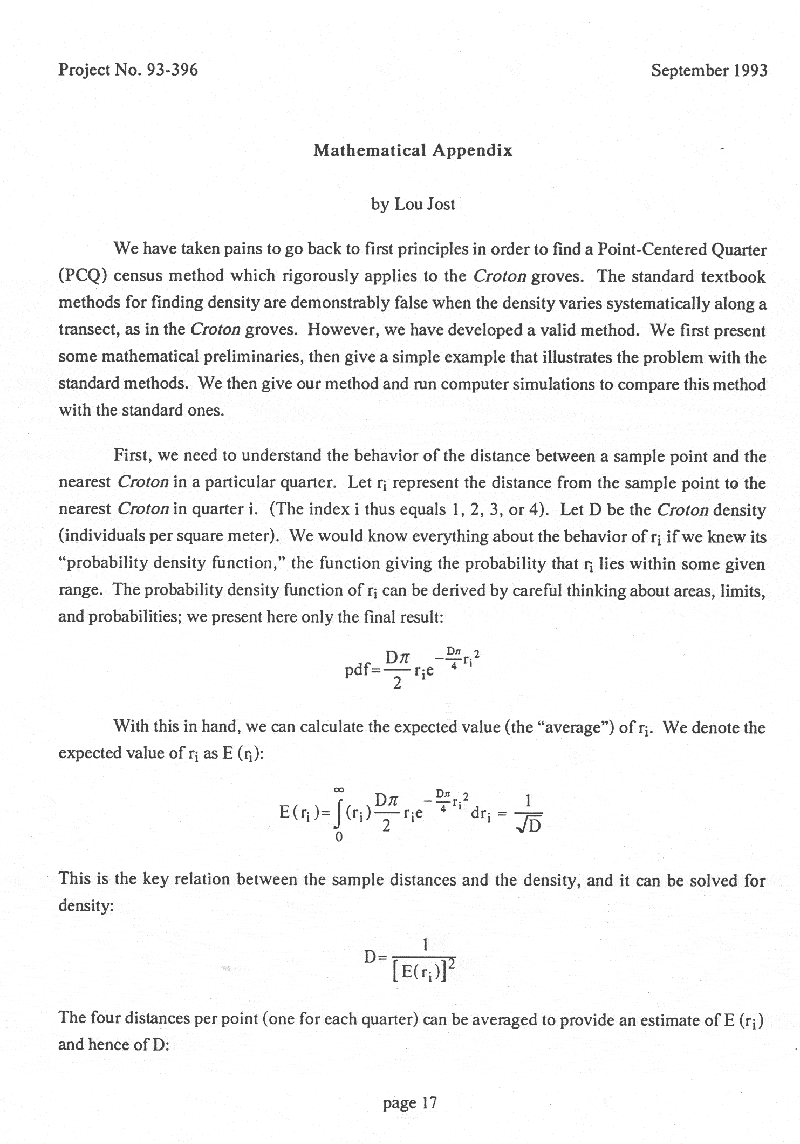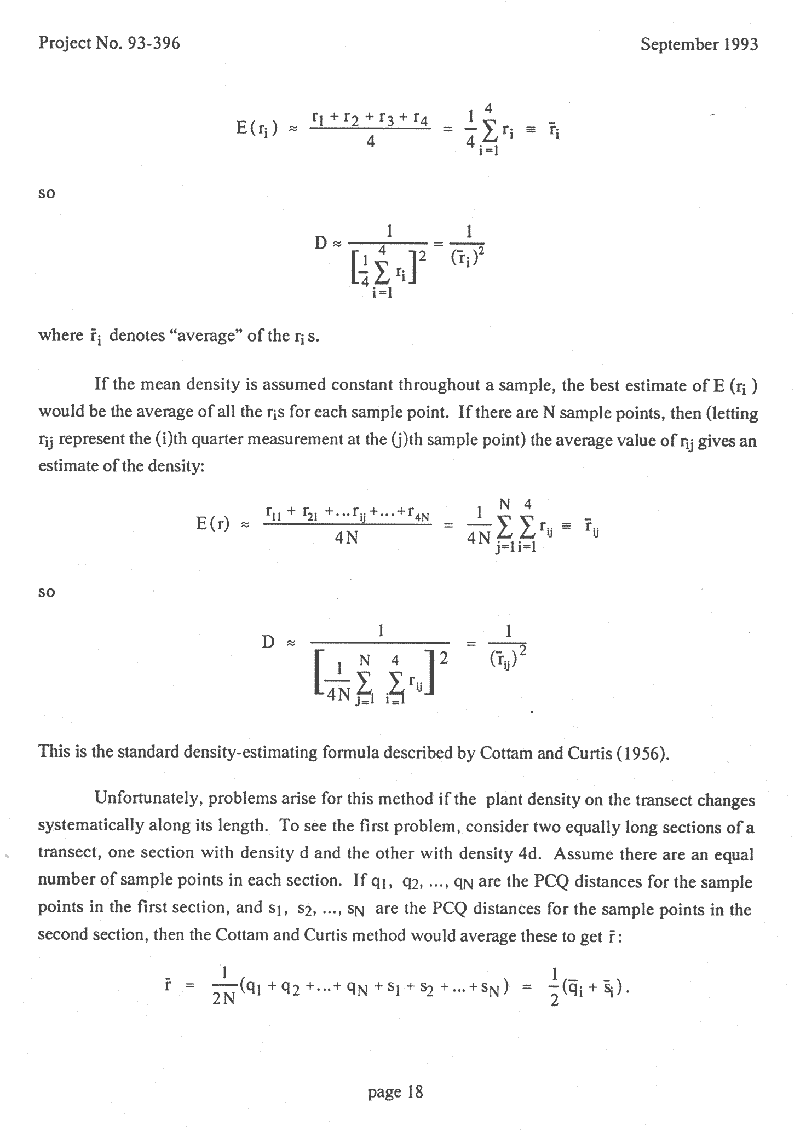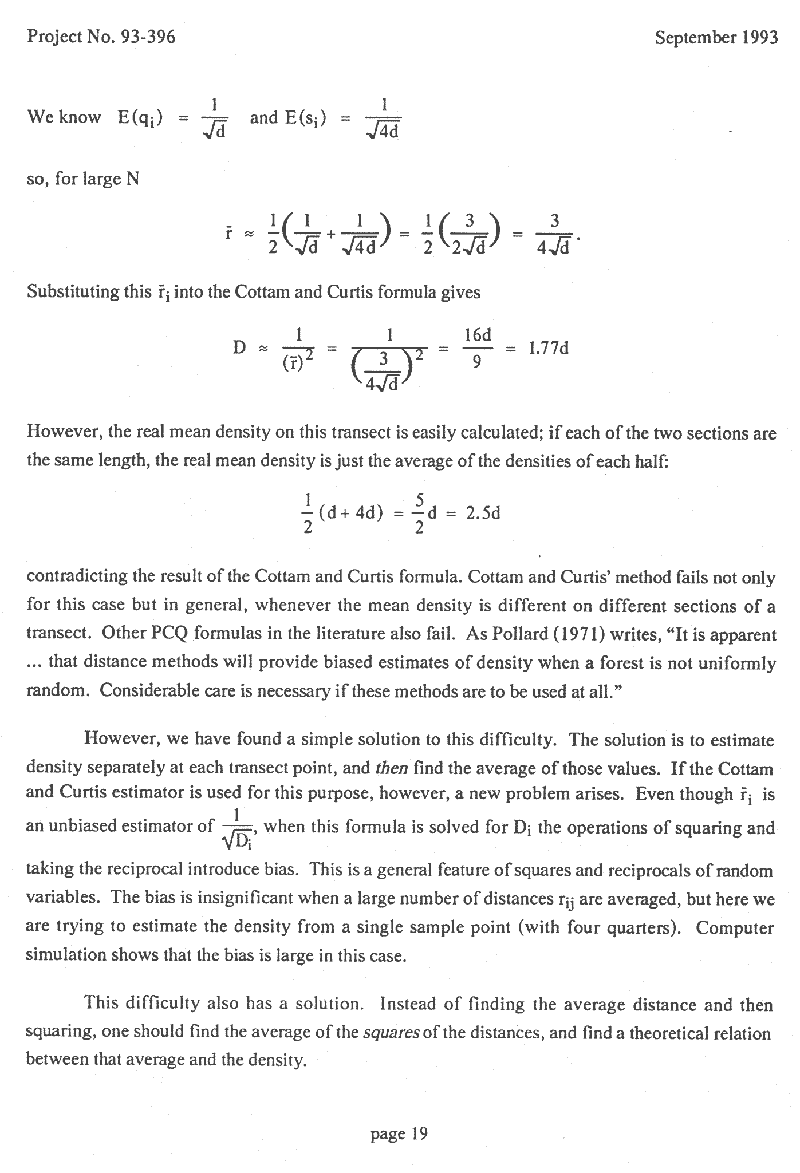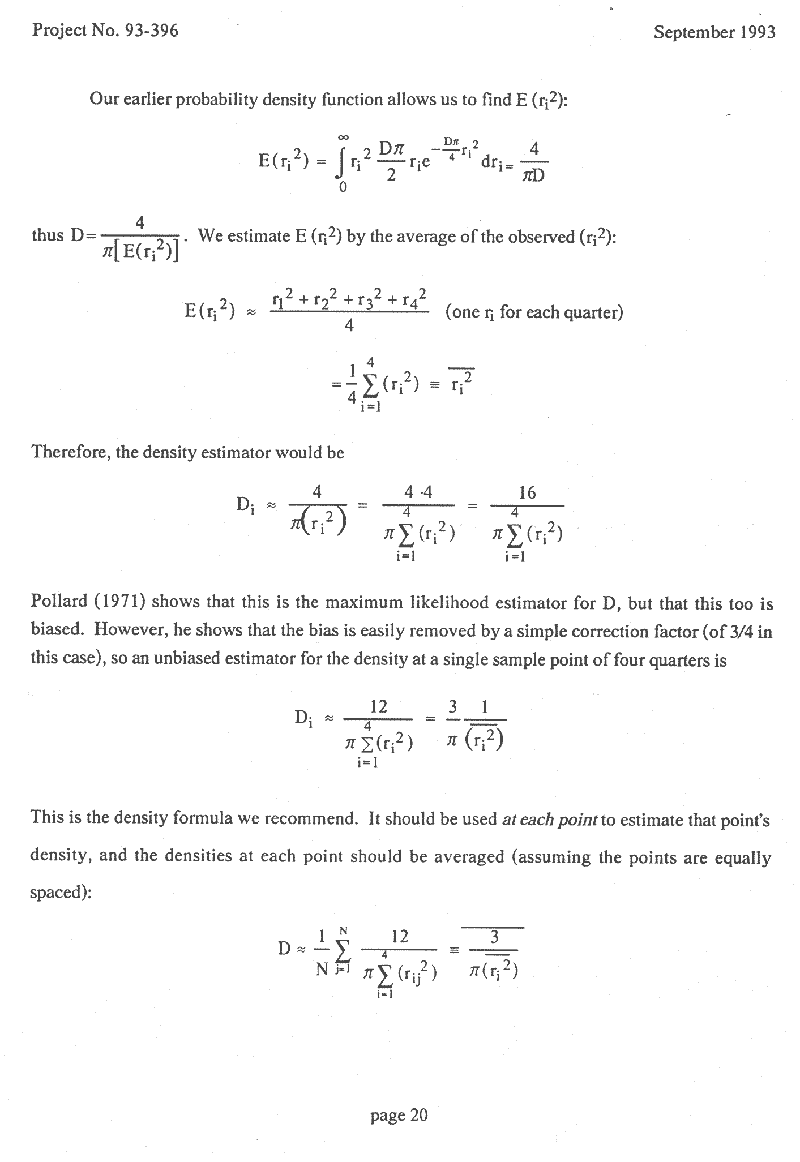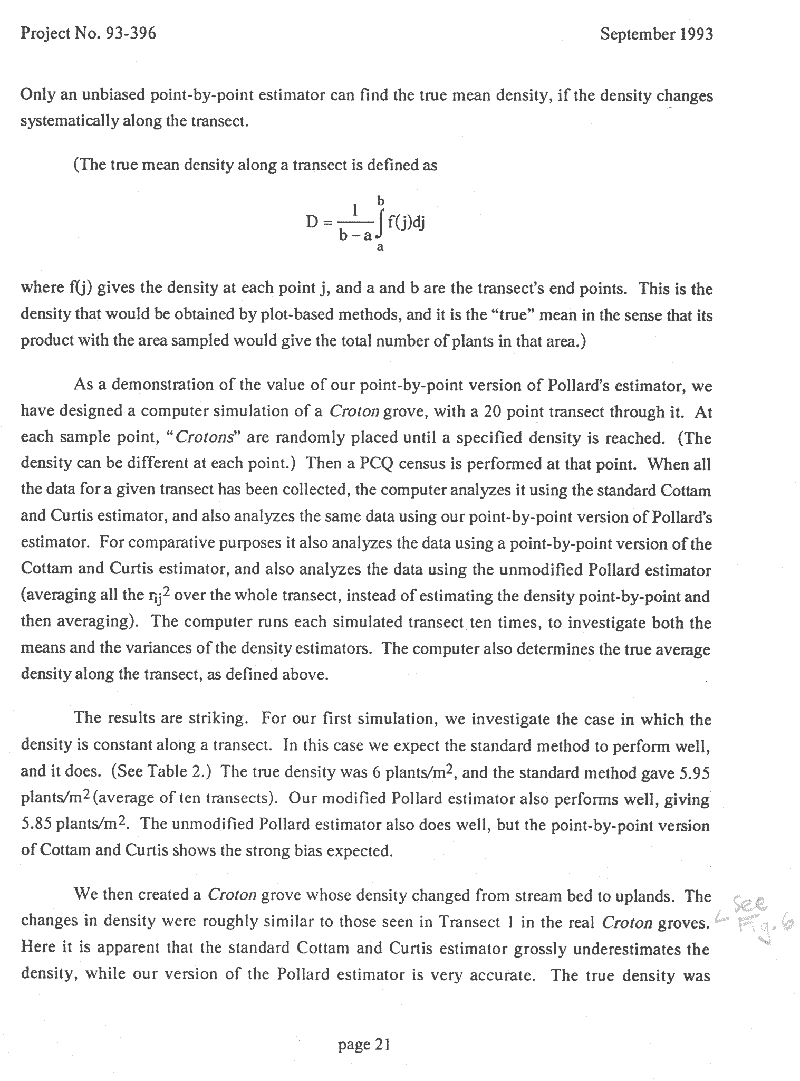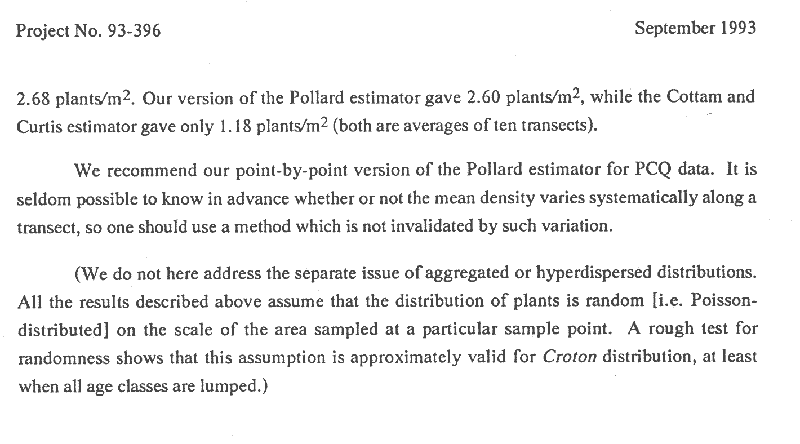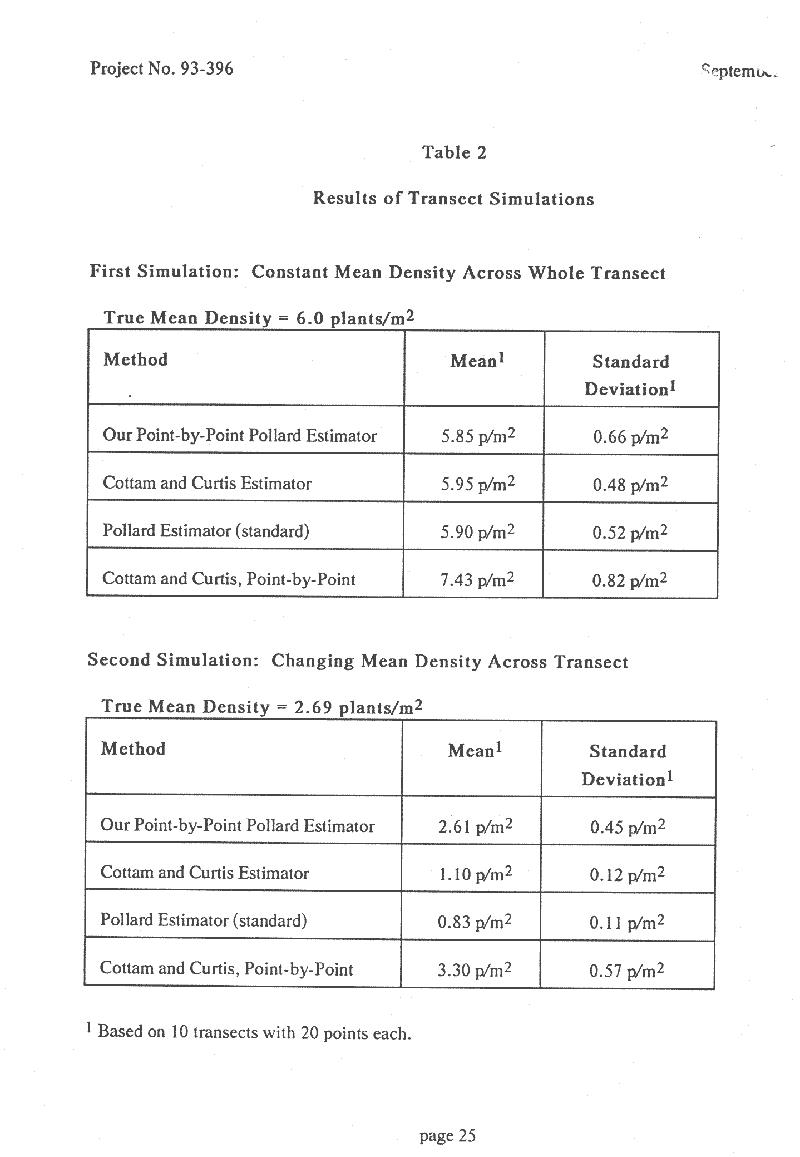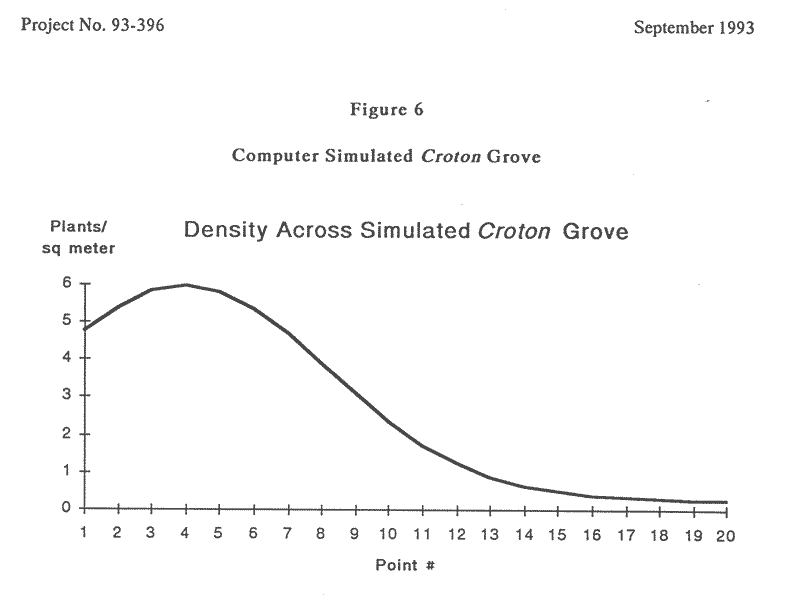A Simple Distance Estimator for Plant Density in Nonuniform Stands
Ho

 This paper resolves some problems with the standard Point Centered Quarter (PCQ) method  of estimating plant density based on distance measurements. The paper is a byproduct of a study of the Texabama Croton (Croton alabamensis var. texensis), a threatened species, done by DLS Associates of Austin, TX.  A density estimate was needed for the croton population on the Fort Hood military base, where a large population was present. The population density varied significantly from place to place, making the standard Point Centered Quarter method inapplicable. I invented a new formula which uses the same data as the PCQ method but combines the data in a way that is not invalidated by systematic variation in density along a transect.      My new formula has been tested both by computer simulations and in the field, and is always more accurate than the standard method when density is not uniform. This new formula should replace the standard PCQ formula in all applications, since plant density is virtually never uniform.     I want to thank my former boss at DLS Associates, David Steed, and my coworker Dan Thompson for their support in the development of this formula.

 A Simple Distance Estimator for Plant Density in Nonuniform Stands By Lou Jost   Abstract      The most widely used distance-based estimates of plant density are not valid for plant stands which exhibit systematic density variations over the study area. A new estimator for use with standard Point Centered Quarter data is presented here which is valid under these conditions. Field tests and computer simulations show the standard estimator to be severely biased, and the new estimator to be accurate, when density varies systematically across a study area.   Introduction    Distance methods for estimating plant density were popularized by Cottam and Curtis (1956) and are widely used. The most common distance method for estimating density is the Point-Centered Quarter (PCQ) method, which estimates density from the relation : 1.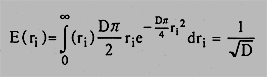where D = mean stand density and ri  is the distance from a sample point to the nearest plant of interest.       This relation is inverted and squared to solve for D in terms of the expectation value of the distance r. These operations introduce statistical bias, which can be large when sample size is small. Moore (1954) showed how to avoid this error by finding the expectation value not of r but of r squared: 2. Inverting to solve for D still introduces bias, but this bias can be calculated explicitly and corrected by a simple multiplicative factor that depends on sample size (Moore 1954, Pollard 1971). Unfortunately the importance of this result has not been appreciated.       The second problem with the standard PCQ estimator, its failure when density varies systematically along the sample transect, has plagued all simple distance estimators. This problem led Pollard (1971) to conclude: "It is apparent that distance methods will provide biased estimates of density when a forest is not uniformly random. Considerable care is necessary if these methods are to be used at all."   A New Robust Density Estimator      There is a simple solution to the above-mentioned difficulty. Moore and Pollard's unbiased density estimator is valid even for very small samples, so their estimator can be applied to the four distance measurements obtained at a single PCQ sampling point. If the points are evenly spaced, the point-by-point results can be averaged to give an unbiased estimate of the overall density: 3. where N is the number of PCQ sampling points and rij is the distance from the jth sampling point to the nearest plant of interest in the ith quadrant. This estimator works even in nonuniform forests, and we propose that it replace the standard Cottam and Curtis formula in all applications.       The standard deviation of this estimator is 4. where bj is the density at the jth point. The relative uncertainty of this estimator (d/D) is independent of mean density and relatively insensitive to the degree of density variation along the sample transect. It is convenient to express the relative uncertainty as 5. The multiplier k achieves its minimum value of 1 when the density is uniform. As long as density varies by less than a factor of two in a stand, k will be less than 1.06. When density varies by a factor of five, k will be less than 1.35, and when density varies by a factor of ten, k will be less than 1.7. Even when density varies a hundredfold along a transect, k can still be small (<1.4) as long as the high-density areas constitute more than half the stand. High values of k (2.5 to 4) are reached when a small proportion (<10%) of the stand has a density 30 to 100 times greater than the rest of the stand.   Tests of the Estimator      The proposed estimator (3) has been tested in computer simulations and field trials, both with excellent results. In a computer simulation of a stand whose density varied tenfold along a transect, twenty evenly spaced points were used to provide PCQ data. The true mean density of the simulated stand was 2.69. The standard PCQ formula of Cottam and Curtis gave a density of 1.1, while the estimator proposed here gave a very accurate density of 2.61 (These densities are averages of ten simulations).    In the field, my colleagues and I studied the distribution of a threatened shrub, Croton alabamensis var. texensis, in Fort Hood, Texas (DLS Associates 1993). Stands of this shrub showed a large systematic variation in density, approximately tenfold in our sample area. We established a transect with 20 evenly spaced points, and we measured the density using the standard PCQ formula and the new formula proposed here. We also measured the density by establishing uniformly spaced plots along the transect and counting all individuals within each plot. The plot-based method gave a density of 3.2, in good agreement with the density calculated by the new estimator proposed here, 2.9. The standard estimator of Cottam and Curtis gave a strikingly inaccurate estimate of .81.   Conclusions      The density estimator proposed here (3) requires no more data collection or calculations than the standard Cottam and Curtis estimator (6), but it is not biased when applied to stands which exhibit systematic variation in density. In contrast, standard estimators are inaccurately low (sometimes by more than a factor of two) when mean density varies significantly along a transect. Natural plant stands are virtually never without density variation, so the estimator proposed here should always be used in preference to the standard formula. With this modification, the PCQ method becomes a powerful and robust tool for quickly measuring the mean density even in highly inhomogeneous stands.    The seperate issues of aggregated or hyperdispersed plant distributions have not been addressed here. The results assume that the plants are distributed approximately randomly (i.e Poisson distributed) on the scale of the area sampled at each sampling point. References Cottam, G., and J. T.Curtis. 1956. The use of distance measures in phytosociological sampling. Ecology 37(3): 451-460. DLS Associates. 1993. Texabama Croton Investigations. DLS Associates, Austin, TX. Moore, P. G. 1954. Spacing in plant populations. Ecology 35: 222-227. Pollard, J. H. 1971. On distance estimators of density in randomly distributed forests. Biometrics 27: 991-1002.